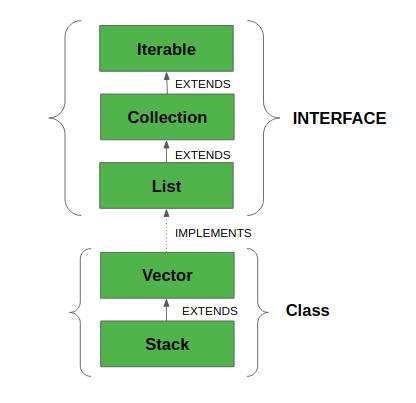Related Articles

# Stack Class in Java

• Difficulty Level : Easy
• Last Updated : 17 Aug, 2020

Java Collection framework provides a Stack class that models and implements a Stack data structure. The class is based on the basic principle of last-in-first-out. In addition to the basic push and pop operations, the class provides three more functions of empty, search, and peek. The class can also be said to extend Vector and treats the class as a stack with the five mentioned functions. The class can also be referred to as the subclass of Vector. The below diagram shows the hierarchy of the Stack classThe class supports one default constructor Stack() which is used to create an empty stack

Declaration:

```public class Stack<E> extends Vector<E>
```

All Implemented Interfaces:

• Serializable: It is a marker interface that classes must implement if they are to be serialized and deserialized.
• Cloneable: This is an interface in Java which needs to be implemented by a class to allow its objects to be cloned.
• Iterable<E>: This interface represents a collection of objects which is iterable — meaning which can be iterated.
• Collection<E>: A Collection represents a group of objects known as its elements. The Collection interface is used to pass around collections of objects where maximum generality is desired.
• List<E>: The List interface provides a way to store the ordered collection. It is a child interface of Collection.
• RandomAccess: This is a marker interface used by List implementations to indicate that they support fast (generally constant time) random access.

### How to Create a Stack?

In order to create a stack, we must import java.util.stack package and use the Stack() constructor of this class. The below example creates an empty Stack.

Stack<E> stack = new Stack<E>();

Here E is the type of Object.

Example:

## Java

 `// Java code for stack implementation`` ` `import` `java.io.*;``import` `java.util.*;`` ` `class` `Test``{   ``    ``// Pushing element on the top of the stack``    ``static` `void` `stack_push(Stack stack)``    ``{``        ``for``(``int` `i = ``0``; i < ``5``; i++)``        ``{``            ``stack.push(i);``        ``}``    ``}``     ` `    ``// Popping element from the top of the stack``    ``static` `void` `stack_pop(Stack stack)``    ``{``        ``System.out.println(``"Pop Operation:"``);`` ` `        ``for``(``int` `i = ``0``; i < ``5``; i++)``        ``{``            ``Integer y = (Integer) stack.pop();``            ``System.out.println(y);``        ``}``    ``}`` ` `    ``// Displaying element on the top of the stack``    ``static` `void` `stack_peek(Stack stack)``    ``{``        ``Integer element = (Integer) stack.peek();``        ``System.out.println(``"Element on stack top: "` `+ element);``    ``}``     ` `    ``// Searching element in the stack``    ``static` `void` `stack_search(Stack stack, ``int` `element)``    ``{``        ``Integer pos = (Integer) stack.search(element);`` ` `        ``if``(pos == -``1``)``            ``System.out.println(``"Element not found"``);``        ``else``            ``System.out.println(``"Element is found at position: "` `+ pos);``    ``}`` ` ` ` `    ``public` `static` `void` `main (String[] args)``    ``{``        ``Stack stack = ``new` `Stack();`` ` `        ``stack_push(stack);``        ``stack_pop(stack);``        ``stack_push(stack);``        ``stack_peek(stack);``        ``stack_search(stack, ``2``);``        ``stack_search(stack, ``6``);``    ``}``}`

Output:

```Pop Operation:
4
3
2
1
0
Element on stack top: 4
Element is found at position: 3

### Performing various operations on Stack class

1. Adding Elements: In order to add an element to the stack, we can use the push() method. This push() operation place the element at the top of the stack.

## Java

 `// Java program to add the``// elements in the stack``import` `java.io.*;``import` `java.util.*;`` ` `class` `StackDemo {``   ` `      ``// Main Method``    ``public` `static` `void` `main(String[] args)``    ``{`` ` `        ``// Default initialization of Stack``        ``Stack stack1 = ``new` `Stack();`` ` `        ``// Initialization of Stack``        ``// using Generics``        ``Stack stack2 = ``new` `Stack();`` ` `        ``// pushing the elements``        ``stack1.push(``4``);``        ``stack1.push(``"All"``);``        ``stack1.push(``"Geeks"``);`` ` `        ``stack2.push(``"Geeks"``);``        ``stack2.push(``"For"``);``        ``stack2.push(``"Geeks"``);`` ` `          ``// Priniting the Stack Elements``        ``System.out.println(stack1);``        ``System.out.println(stack2);``    ``}``}`

Output:

```[4, All, Geeks]
[Geeks, For, Geeks]
```

2. Accessing the Element: To retrieve or fetch the first element of the Stack or the element present at the top of the Stack, we can use peek() method. The element retrieved does not get deleted or removed from the Stack.

## Java

 `// Java program to demonstrate the accessing``// of the elements from the stack``import` `java.util.*;``import` `java.io.*;`` ` `public` `class` `StackDemo {`` ` `      ``// Main Method``    ``public` `static` `void` `main(String args[])``    ``{``        ``// Creating an empty Stack``        ``Stack stack = ``new` `Stack();`` ` `        ``// Use push() to add elements into the Stack``        ``stack.push(``"Welcome"``);``        ``stack.push(``"To"``);``        ``stack.push(``"Geeks"``);``        ``stack.push(``"For"``);``        ``stack.push(``"Geeks"``);`` ` `        ``// Displaying the Stack``        ``System.out.println(``"Initial Stack: "` `+ stack);`` ` `        ``// Fetching the element at the head of the Stack``        ``System.out.println(``"The element at the top of the"``                           ``+ ``" stack is: "` `+ stack.peek());`` ` `        ``// Displaying the Stack after the Operation``        ``System.out.println(``"Final Stack: "` `+ stack);``    ``}``}`

Output:

```Initial Stack: [Welcome, To, Geeks, For, Geeks]
The element at the top of the stack is: Geeks
Final Stack: [Welcome, To, Geeks, For, Geeks]```

3. Removing Elements: To pop an element from the stack, we can use the pop() method. The element is popped from the top of the stack and is removed from the same.

## Java

 `// Java program to demonstrate the removing``// of the elements from the stack``import` `java.util.*;``import` `java.io.*;`` ` `public` `class` `StackDemo {``    ``public` `static` `void` `main(String args[])``    ``{``        ``// Creating an empty Stack``        ``Stack stack = ``new` `Stack();`` ` `        ``// Use add() method to add elements``        ``stack.push(``10``);``        ``stack.push(``15``);``        ``stack.push(``30``);``        ``stack.push(``20``);``        ``stack.push(``5``);`` ` `        ``// Displaying the Stack``        ``System.out.println(``"Initial Stack: "` `+ stack);`` ` `        ``// Removing elements using pop() method``        ``System.out.println(``"Popped element: "``                           ``+ stack.pop());``        ``System.out.println(``"Popped element: "``                           ``+ stack.pop());`` ` `        ``// Displaying the Stack after pop operation``        ``System.out.println(``"Stack after pop operation "``                           ``+ stack);``    ``}``}`

Output:

```Initial Stack: [10, 15, 30, 20, 5]
Popped element: 5
Popped element: 20
Stack after pop operation [10, 15, 30]```

### Methods inherited from class java.util.Vector

Note: Please note that the Stack class in Java is a legacy class and inherits from Vector in Java. It is a thread-safe class and hence involves overhead when we do not need thread safety. It is recommended to use ArrayDeque for stack implementation as it is more efficient in a single-threaded environment.

## Java

 `// A Java Program to show implementation``// of Stack using ArrayDeque`` ` `import` `java.util.*;`` ` `class` `GFG {``    ``public` `static` `void` `main (String[] args) {``        ``Deque stack = ``new` `ArrayDeque();``        ``stack.push(``'A'``);``        ``stack.push(``'B'``);``        ``System.out.println(stack.peek());``        ``System.out.println(stack.pop());``    ``}``}`

Output:

```B
B```

Attention reader! Don’t stop learning now. Get hold of all the important DSA concepts with the DSA Self Paced Course at a student-friendly price and become industry ready.  To complete your preparation from learning a language to DS Algo and many more,  please refer Complete Interview Preparation Course.

In case you wish to attend live classes with experts, please refer DSA Live Classes for Working Professionals and Competitive Programming Live for Students.

My Personal Notes arrow_drop_up